# Pronúncia de vogais e semi-vogais

• Nota de Estudos
• Rever Tópicos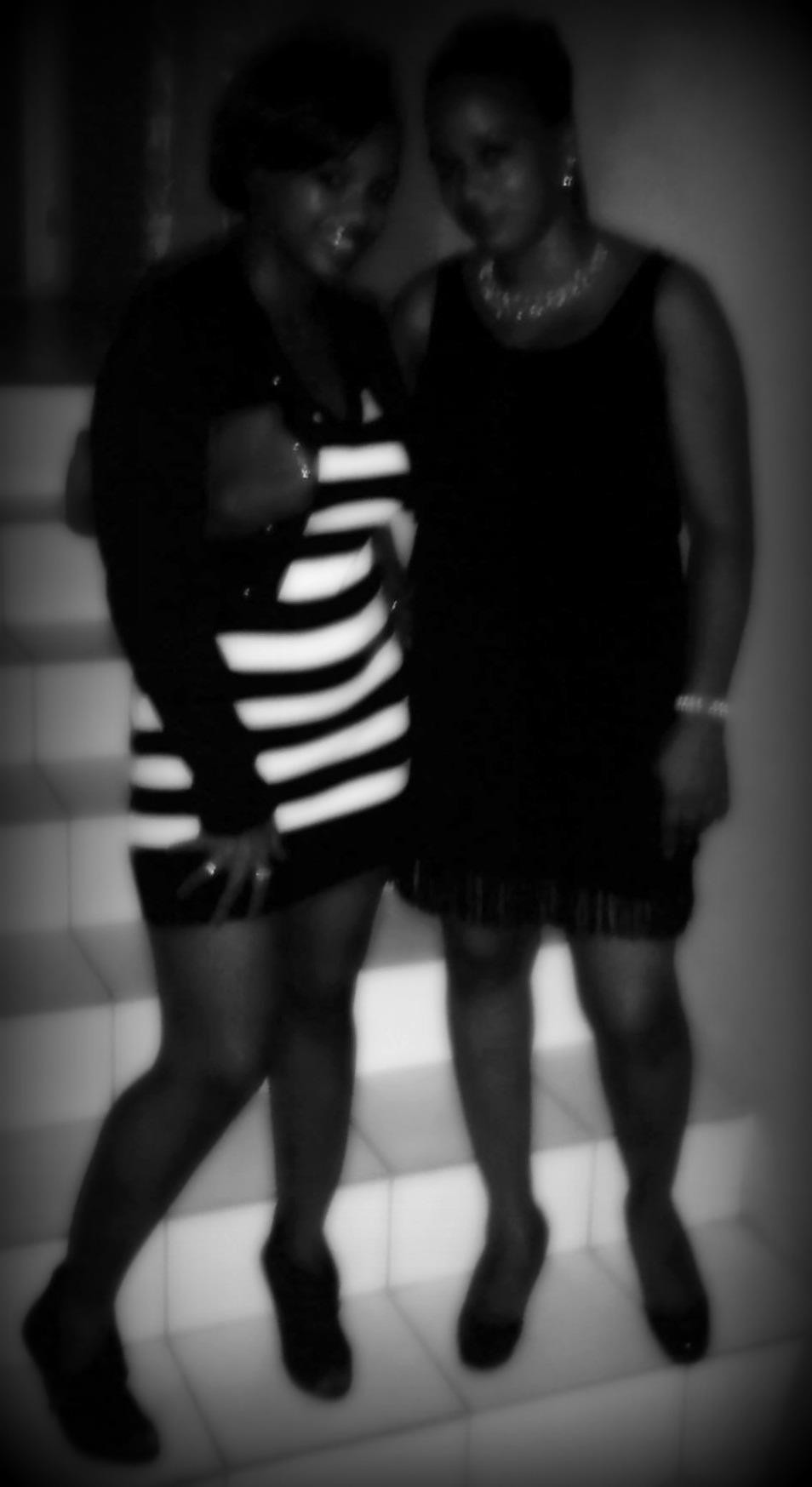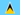Philippa D. 1 0 The site seems to be experiencing a problem as I am only able to hear the french but not able to see the words. Please help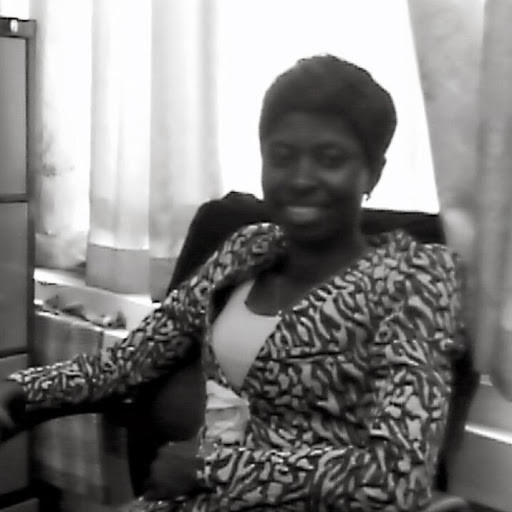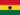Helina Afia G. 1 0 can't access the note pls helpSylvester K. 1 0 can we have a document to help revision? can the module made more interactive?Gloria A. 1 0 interactive videos?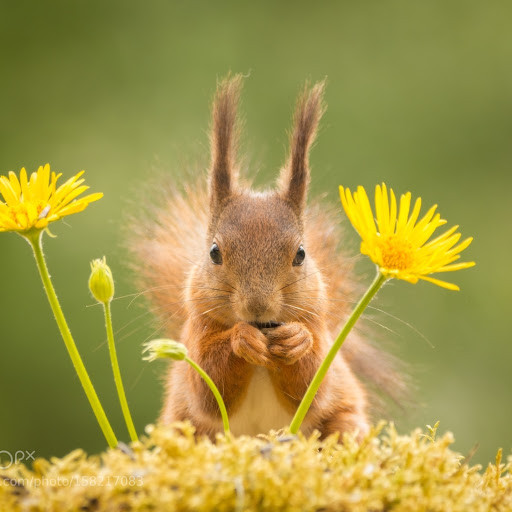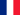OooL. L. 4 0 There's a pronounciation difference between "a" and "â", but as a beginner, you don't have to bother.OooL. L. 3 0 (masculin: m, féminin: f), le papa (m)= daddy. le garage (m) = garage. l'âge (m) = age. le câble (m) = cable (wire). de = from/of. cela = this/it. ledéfilé (m) = march/procession. parler = to speak/talk. manger = to eat. le nez (m) = nose. la mère (f) = mother. près = near. la fête (f) = party. la grêle (f) = hail. le ballet, le cabaret. merci = thanks. le sel = salt. évidemment = obviously. récemment = recently. la laine = wool. mais = but. la neige = snow. la reine = queen. l'ami (m) = friend. l'image (f). l'île (f) = island. l'îlot (m) = small island. le cycle. la porte = door. la bosse = hump. rose = rose/pink. la rose = rose. le rose = pink. le mot = word. la côte = coast. drôle (m,f)= funny. la gauche = left. l'autruche (f) = ostrich. l'eau (f) = water. beau (m) = beautiful. le bateau = boat. deux = 2. le feu = fire. l'heure (f) = hour. le meuble = piece of furniture. l’œuf (m) = egg. vœux (m) = wish. la sœur = sister. le fou = crazy. le bijou = jewel. tu = you. toi = you (pronoun), le mur = wall. la flûte = flute. brûler = to burn. l'oiseau (m) = bird. lui = him. l'huile (f) = oil. naïf (m) = naive. haïr = to hate. la typhoïde, la thyroïde. le pied = foot. le lieu =place/location. There's a spelling mistake for yo-yo, it's spelled yoyo in French. le yoyo, le yoga. l'orteil (m) = toe. le pareil= same. l'abeille (f) = bee. la vieille = old. l'écureuil (m) = squirrel. le deuil = mourning. la feuille = leave/sheet. l’œil (m) = eye. oui = yes. Louis = Lewis. le fenouil = fennel. la ratatouille (dish). la rouille = rust.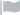Wajih Z. 2 0 évidemment beauWajih Z. 0 0 toi parler en françaisWajih Z. 0 0 merci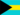Sheala F. 1 0 A Pdf Format would really assist, am not sure I will remember everything.### Você recebeu uma nova notificação

Clique aqui para visualizar todos eles